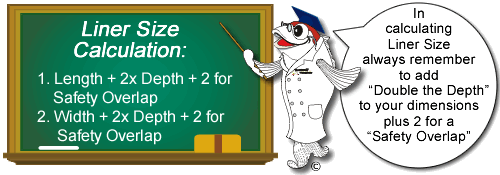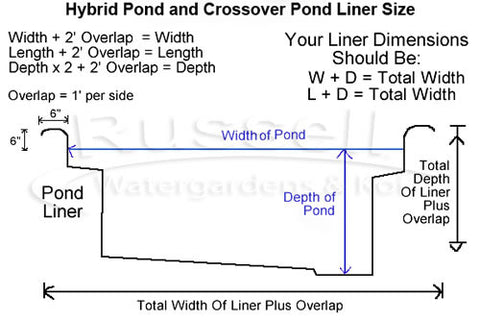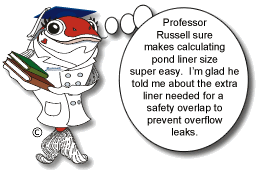#How to Calculate Pond Liner Size

## Water Garden Pond Liner Size

To calculate your pond liner size for a water garden pond:

• Add Safety Overlap of 1' on each side of pond - or simply 2' to each dimension.

•Example:

Your Water Garden Pond is going to be 17' long by 12' feet wide, with a maximum depth of 2'.

Add "Double your Depth" to each dimension to accommodate the vertical walls in the pond. (2 x 2 = 4) So the dimensions adjusted for depth are now 21' long and 16' wide. (17 + 4 = 21 and 12 + 4 = 16)

Now add a "Safety Overlap" of 2' to each of those dimensions. (21 + 2 = 23, and 16 + 2 = 18). Your 17' long by 12' wide pond with a 2' depth and 1' of Safety Overlap on each side would need a 23' x 18' liner.

Liner is sold in 5' increments - so you would purchase a 25' x 20' liner for this pond.

Another example:
11'l x 8'w x 2'd Water Garden Pond
(2' depth x 2 = 4)

Add double the depth to each dimension and add 2' of Safety Overlap to each dimension.
11 + 4 = 15. For overlap add; 15 + 2 = 17 total length.
8 + 4 = 12. For overlap add; 12 + 2 = 14 total width.

Purchase a 20' x 15' Liner for an 11' x 8' pond @ 2' deep.

##Hybrid Pond/Crossover Pond Liner Size

To calculate your pond liner size for a hybrid pond or a crossover pond:

• Add Safety Overlap of 1' on each side of pond - or simply 2' to each dimension.

•Example:
Your Hybrid Pond™ is going to be 14' long by 11' feet wide, with a maximum depth of 3'.

Add "Double your Depth" to each dimension to accommodate the vertical walls in the pond. (3 x 2 = 6) So the dimensions adjusted for depth are now 20' long and 17' wide. (14 + 6 = 20 and 11 + 6 = 17)

Now add a "Safety Overlap" of 2' to each of those dimensions. (20 + 2 = 22, and 17 + 2 = 19). Your 14' long by 11' wide pond with a 3' depth and 1' of Safety Overlap on each side would need a 22' x 19' liner.

Liner is sold in 5' increments - so you would purchase a 25' x 20' liner for this pond.

Another example:
18'l x 14'w x 4'd hybrid pond or crossover pond
(4' depth x 2 = 8)

Add double the depth to each dimension and add 2' of Safety Overlap to each dimension.
18 + 8 = 26.For overlap add; 26 + 2 = 28 total length.
14 + 8 = 22. For overlap add; 22 + 2 = 24 total width.

Purchase a 30' x 25' Liner for an 18' x 14' pond @ 4' deep.

##Koi Pond Liner Size

To calculate your pond liner size for a hybrid pond or a crossover pond:

• Add Safety Overlap of 1' on each side of pond - or simply 2' to each dimension.

•Example:
Your Koi Pond is going to be 14' long by 10' feet wide, with a maximum depth of 5'.

Add "Double your Depth" to each dimension to accommodate the vertical walls in the pond. (5 x 2 = 10) So the dimensions adjusted for depth are now 24' long and 20' wide. (14 + 10 = 24 and 10 + 10 = 20)

Now add a "Safety Overlap" of 2' to each of those dimensions. (24 + 2 = 26, and 20 + 2 = 22). Your 14' long by 11' wide pond with a 5' depth and 1' of Safety Overlap on each side would need a 26' x 22' liner.

Liner is sold in 5' increments - so you would purchase a 30' x 25' liner for this pond.

Another example:
32'l x 21'w x 6'd Koi Pond
(6' depth x 2 = 12)Add double the depth to each dimension and add 2' of Safety Overlap to each dimension.
32 + 12 = 44. For overlap add; 44 + 2 = 46 total length.
21 + 12 = 33. For overlap add; 33 + 2 = 35 total width.

Purchase a 50' x 35' Liner for a 32' x 21' pond @ 6' deep.

Top of Page